#### How to calculate Standard Deviation?

Standard deviation is a statistical measure of how spread out a set of data is from the mean. It is calculated by taking the square root of the variance, which is the average of the squared deviations from the mean.

## Why is standard deviation important?

Standard deviation can be used to compare different sets of data, or to identify outliers in a set of data. It can also be used to calculate confidence intervals and to assess the significance of statistical tests.

## How to calculate standard deviation in Excel

There are two ways to calculate standard deviation in Excel: using the STDEV function and using the Data Analysis ToolPak.

## Using the STDEV function

The STDEV function calculates the standard deviation, which measures the spread or dispersion of a set of data points.

Let’s start by preparing your data. From this data, we will see how to calculate the Standard Deviation here.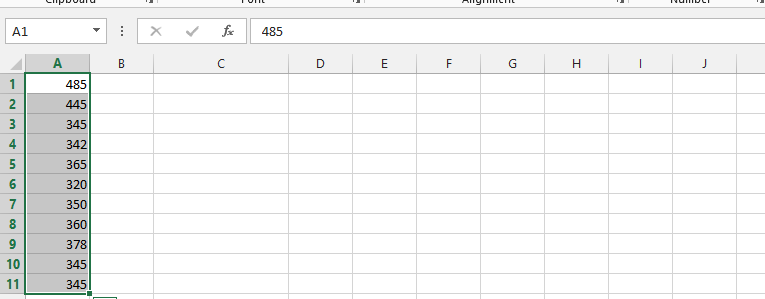Click on a blank column and type in =STDEV(choose all columns with numbers in them, ex. A1:A11), and then press enter.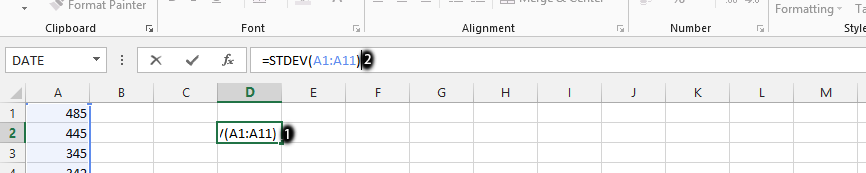The Standard Deviation is calculated.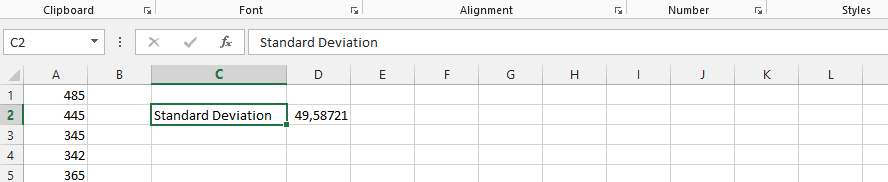Simple and quick. Luckily, Excel has a dedicated STDEV function for Standard Deviation calculations. There are also other Excel functions:

• STDEV.P: Standard deviation of the population.
• STDEV.S: Standard deviation of the sample.
• STDEVA: Sample standard deviation, which does not ignore logical values.
• STDEVPA: Standard deviation of the population that does not ignore logical values.

## Using the Data Analysis ToolPak

Let’s calculate the Standard Deviation using the Data Analysis ToolPak Add-In.

First, you need to make sure your Data Analysis Toolpak Add-In is installed. Here is a lesson on how to install the Data Analysis Toolpak Add-In.

Navigate to the Data Tab on the Ribbon. Click the Data Analysis button.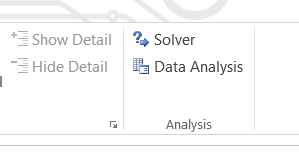A new window appears. Choose Descriptive Statistics and click OK.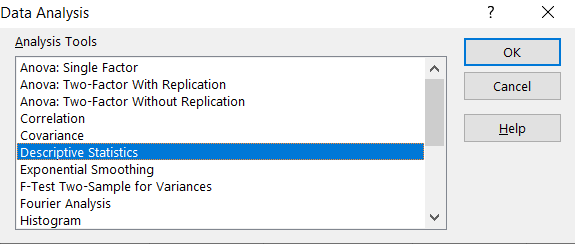Enter the proper parameters:

• Input Range: Your data range (\$A\$1:\$A\$11 in my example).
• Labels in first row: There are no labels in my data table.
• Output Range: Choose where you want to get your standard deviation calculated (I picked the same Sheet \$F\$1:\$G\$14).
• Summary statistics: Make sure it is checked.

Click “OK.” Excel will generate a descriptive statistics table that includes the standard deviation of the data.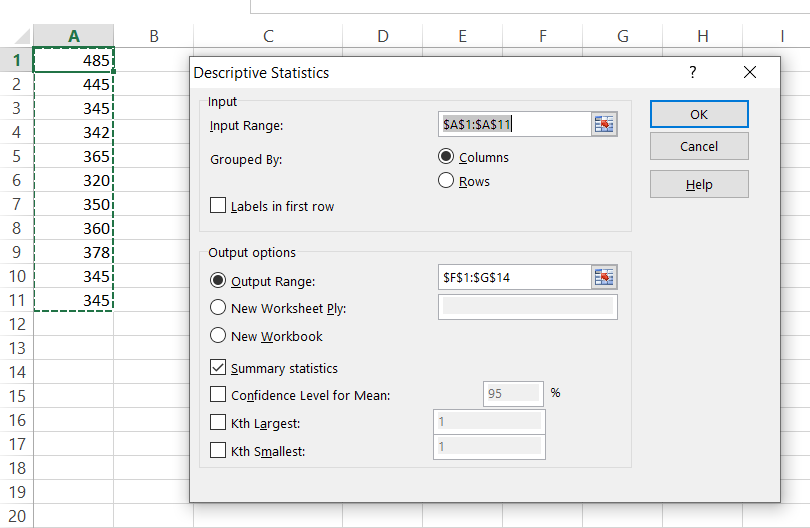And this is how you can see my statistics summary. The Standard Deviation is calculated in row 7. The result is the same as in Example 1, where we checked how to calculate the Standard Deviation using the STDEV Excel function.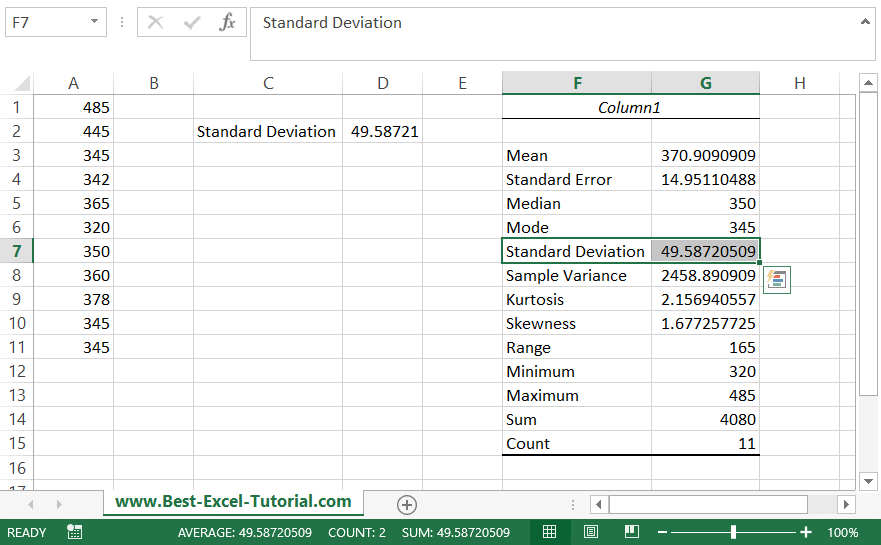Note that the standard deviation is sensitive to outliers, so it’s important to carefully review your data and make sure it’s accurate before calculating the standard deviation.

## Which method should I use?

The STDEV function is the quickest and easiest way to calculate standard deviation in Excel. However, if you need to calculate standard deviation for a large data set or if you need to include other summary statistics in the output, you may want to use the Data Analysis ToolPak.

```Further reading:
how to calculate standard deviation in python pandas
```